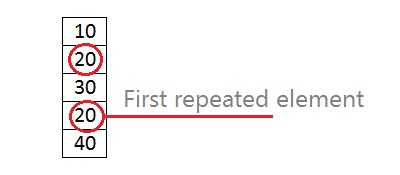Q:

# C program to find the first repeated element in an array

This program will read an integer one dimensional array and find the first repeated element. Let suppose there are 5 elements in an array 10, 20, 30, 20, 40. Here element 20 repeated at 3rd index (count starts from 0).## Program to find first repeated element in C

``````#include <stdio.h>

int main()
{
int arr;
int i,j,n=5;
int ind,ele; //to store index & element

for(i=0; i<n; i++)
{
printf("Enter element %d: ",i+1);
scanf("%d",&arr[i]);
}

printf("Array elements are: ");
for(i=0; i<n; i++)
printf("%d ",arr[i]);

printf("\n");
ind=-1;
//check first repeated element
for(i=0; i<n; i++)
{
for(j=i+1; j<n; j++)
{
if(arr[i]==arr[j])
{
ele=arr[j];
ind=j;
break;
}
}

if(ind != -1)
break;
}
if(ind!=-1)
printf("%d repeated @ %d index\n",ele,ind);
else
printf("There is no repeated element\n");

return 0;
}``````

Output

```Firsr run:
Enter element 1: 10
Enter element 2: 20
Enter element 3: 30
Enter element 4: 20
Enter element 5: 40
Array elements are: 10 20 30 20 40
20 repeated @ 3 index

Second run:
Enter element 1: 10
Enter element 2: 20
Enter element 3: 30
Enter element 4: 40
Enter element 5: 50
Array elements are: 10 20 30 40 50
There is no repeated element ```